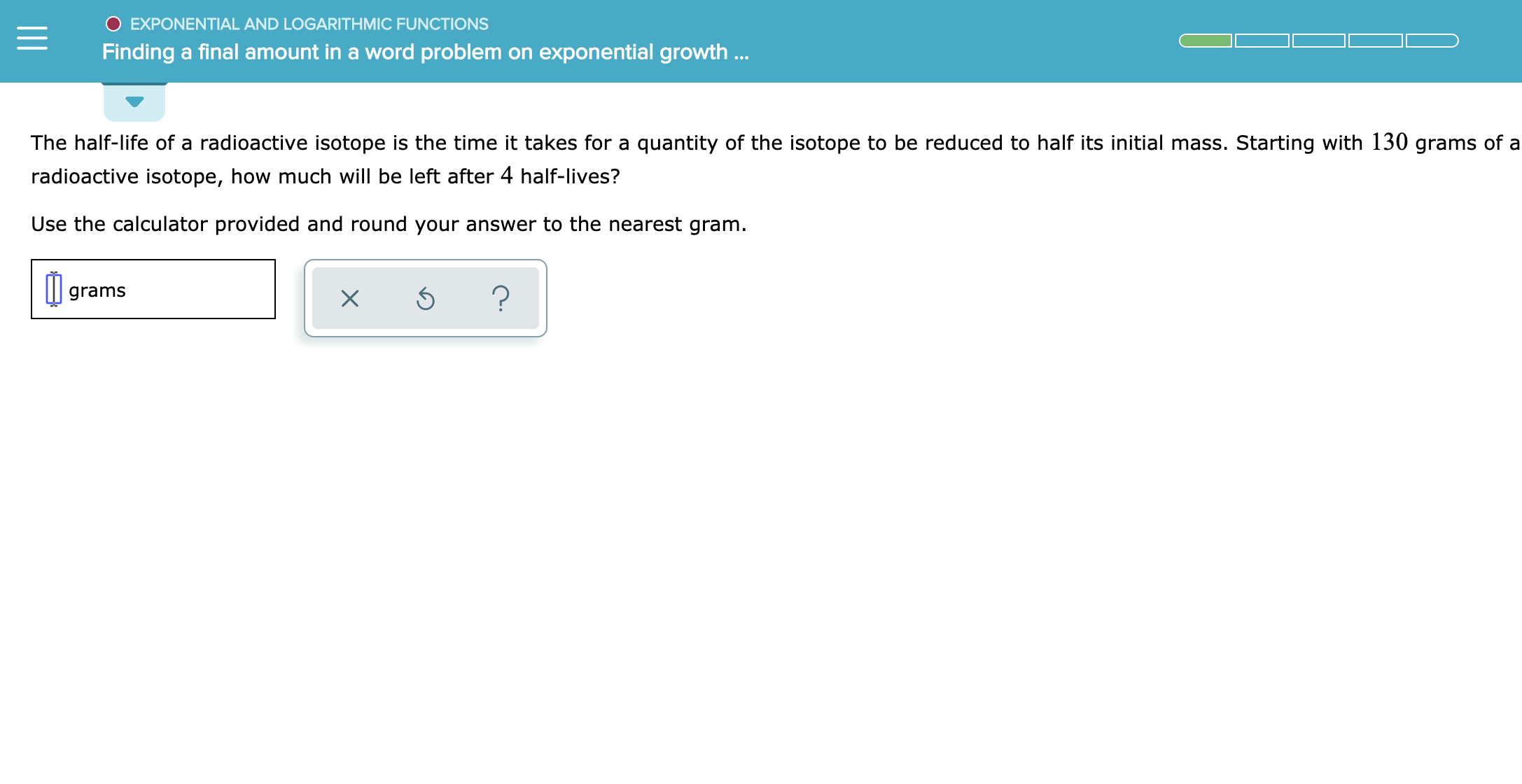# EXPONENTIAL AND LOGARITHMIC FUNCTIONSFinding a final amount in a word problem on exponential growth ...The half-life of a radioactive isotope is the time it takes for a quantity of the isotope to be reduced to half its initial mass. Starting with 130 grams of aradioactive isotope, how much will be left after 4 half-lives?Use the calculator provided and round your answer to the nearest gram.?gramsX

Question
3 views

see attachmenthelp_outlineImage TranscriptioncloseEXPONENTIAL AND LOGARITHMIC FUNCTIONS Finding a final amount in a word problem on exponential growth ... The half-life of a radioactive isotope is the time it takes for a quantity of the isotope to be reduced to half its initial mass. Starting with 130 grams of a radioactive isotope, how much will be left after 4 half-lives? Use the calculator provided and round your answer to the nearest gram. ? grams X fullscreen
check_circle

Step 1

To determine the amount of the radioactive isotope with starting amount 130 grams present after 4 half-lives.

Step 2

The half life of a substance is time after which the initial amount of the substance is reduced to half.

Step 3

Consider that the initial amount of the radioactive substance present is 130 grams.

After 1 half-life the amount of radioactive substance present = 130/2 = 65 grams

After 2 half-life the amount of radioactive substance pre...

### Want to see the full answer?

See Solution

#### Want to see this answer and more?

Solutions are written by subject experts who are available 24/7. Questions are typically answered within 1 hour.*

See Solution
*Response times may vary by subject and question.
Tagged in

### Other